# How to colorize black & white photos with just 100 lines of neural network code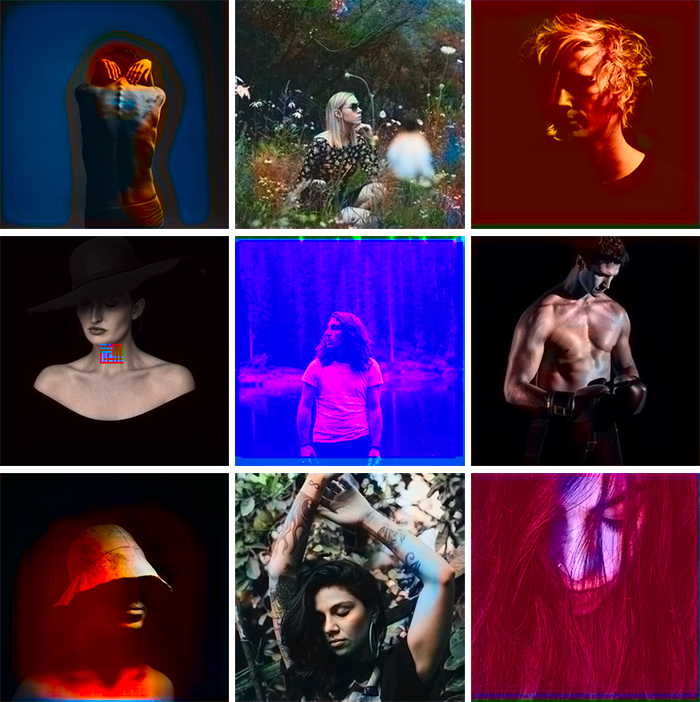The original b&w images are from Unsplash

# Core logic

In this section, I’ll outline how to render an image, the basics of digital colors, and the main logic for our neural network.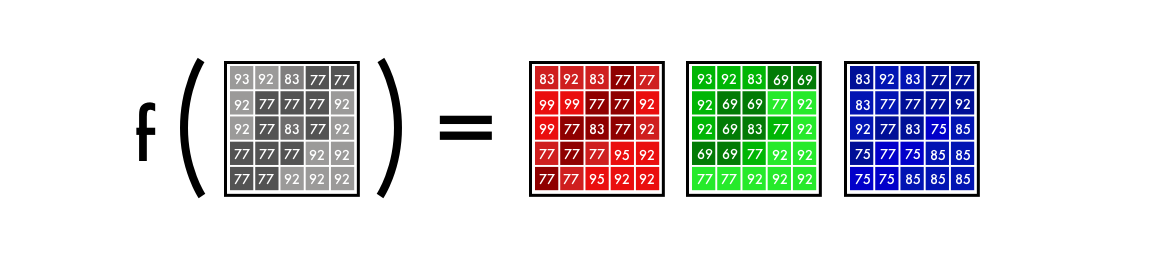f() is the neural network, [B&W] is our input, and [R],[G],[B] is our output.

# Alpha version

We’ll start by making a simple version of our neural network to color an image of a woman’s face. This way, you can get familiar with the core syntax of our model as we add features to it.

## Color space

First, we’ll use an algorithm to change the color channels, from RGB to Lab. L stands for lightness, and a and b for the color spectra green–red and blue–yellow.

## From B&W to color

Our final prediction looks like this. We have a grayscale layer for input, and we want to predict two color layers, the ab in Lab. To create the final color image we’ll include the L/grayscale image we used for the input. The result will be creating a Lab image.

## Run the code on FloydHub

Click here to open a Workspace on FloydHub where you will find the same environment and dataset used for the Full version.

## Alpha version

Once FloydHub is installed, use the following commands:

`git clone https://github.com/emilwallner/Coloring-greyscale-images-in-Keras`
`cd Coloring-greyscale-images-in-Keras/floydhubfloyd init colornet`
`floyd init colornet`
`floyd run --data emilwallner/datasets/colornet/2:data --mode jupyter --tensorboard`
• We mounted a public dataset on FloydHub (which I’ve already uploaded) at the `data`directory with the below line:
`--dataemilwallner/datasets/colornet/2:data`
• We enabled Tensorboard with `--tensorboard`
• We ran the job in Jupyter Notebook mode with `--mode jupyter`
• If you have GPU credit, you can also add the GPU flag `--gpu`to your command. This will make it approximately 50x faster
`floydhub/Alpha version/working_floyd_pink_light_full.ipynb`
`model.fit(x=X, y=Y, batch_size=1, epochs=1)`
`# Get imagesimage = img_to_array(load_img('woman.png'))image = np.array(image, dtype=float)# Import map images into the lab colorspaceX = rgb2lab(1.0/255*image)[:,:,0]Y = rgb2lab(1.0/255*image)[:,:,1:]Y = Y / 128X = X.reshape(1, 400, 400, 1)Y = Y.reshape(1, 400, 400, 2)# Building the neural networkmodel = Sequential()model.add(InputLayer(input_shape=(None, None, 1)))model.add(Conv2D(8, (3, 3), activation='relu', padding='same', strides=2))model.add(Conv2D(8, (3, 3), activation='relu', padding='same'))model.add(Conv2D(16, (3, 3), activation='relu', padding='same'))model.add(Conv2D(16, (3, 3), activation='relu', padding='same', strides=2))model.add(Conv2D(32, (3, 3), activation='relu', padding='same'))model.add(Conv2D(32, (3, 3), activation='relu', padding='same', strides=2))model.add(UpSampling2D((2, 2)))model.add(Conv2D(32, (3, 3), activation='relu', padding='same'))model.add(UpSampling2D((2, 2)))model.add(Conv2D(16, (3, 3), activation='relu', padding='same'))model.add(UpSampling2D((2, 2)))model.add(Conv2D(2, (3, 3), activation='tanh', padding='same'))# Finish modelmodel.compile(optimizer='rmsprop',loss='mse')#Train the neural networkmodel.fit(x=X, y=Y, batch_size=1, epochs=3000)print(model.evaluate(X, Y, batch_size=1))# Output colorizationsoutput = model.predict(X)output = output * 128canvas = np.zeros((400, 400, 3))canvas[:,:,0] = X[:,:,0]canvas[:,:,1:] = outputimsave("img_result.png", lab2rgb(canvas))imsave("img_gray_scale.png", rgb2gray(lab2rgb(canvas)))`
`floyd run --data emilwallner/datasets/colornet/2:data --mode jupyter --tensorboard`

## Technical explanation

To recap, the input is a grid representing a black and white image. It outputs two grids with color values. Between the input and output values, we create filters to link them together. This is a convolutional neural network.

`X = rgb2lab(1.0/255*image)[:,:,0]Y = rgb2lab(1.0/255*image)[:,:,1:]`
`Y = Y / 128`
`output = model.predict(X)output = output * 128`
`canvas = np.zeros((400, 400, 3))canvas[:,:,0] = X[:,:,0]canvas[:,:,1:] = output`

## Takeaways from the Alpha version

• Reading research papers is challenging. Once I summarized the core characteristics of each paper, it became easier to skim papers. It also allowed me to put the details into a context.
• Starting simple is key. Most of the implementations I could find online were 2–10K lines long. That made it hard to get an overview of the core logic of the problem. Once I had a barebones version, it became easier to read both the code implementation, and also the research papers.
• Explore public projects. To get a rough idea for what to code, I skimmed 50–100 projects on colorization on Github.
• Things won’t always work as expected. In the beginning, it could only create red and yellow colors. At first, I had a Relu activation function for the final activation. Since it only maps numbers into positive digits, it could not create negative values, the blue and green spectrums. Adding a tanh activation function and mapping the Y values fixed this.
• Understanding > Speed. Many of the implementations I saw were fast but hard to work with. I chose to optimize for innovation speed instead of code speed.

# Beta version

To understand the weakness of the alpha version, try coloring an image it has not been trained on. If you try it, you’ll see that it makes a poor attempt. It’s because the network has memorized the information. It has not learned how to color an image it hasn’t seen before. But this is what we’ll do in the beta version. We’ll teach our network to generalize.

## The feature extractor

Our neural network finds characteristics that link grayscale images with their colored versions.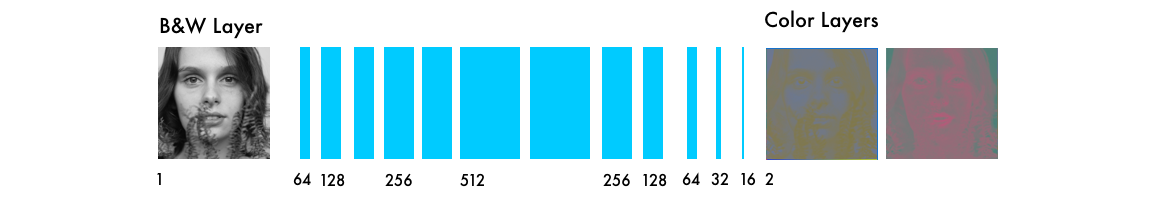The number of filtered images for each step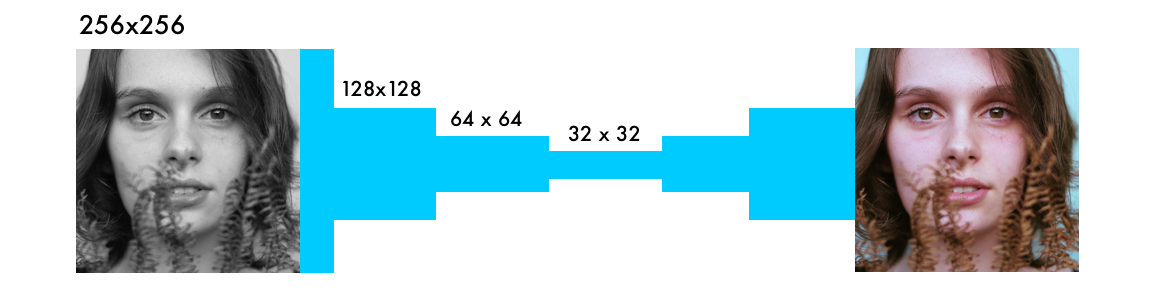We decrease the size in three steps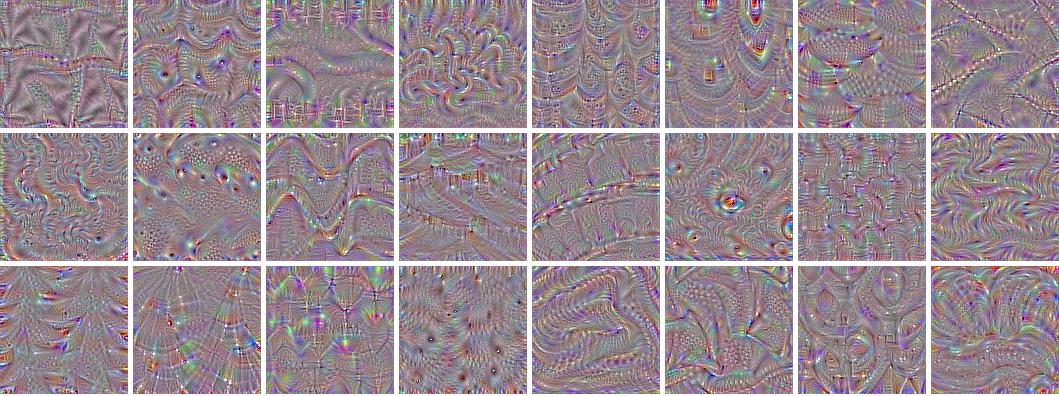From Keras layer tutorial

## From feature extraction to color

The neural network operates in a trial and error manner. It first makes a random prediction for each pixel. Based on the error for each pixel, it works backward through the network to improve the feature extraction.

`# Get imagesX = []for filename in os.listdir('../Train/'):    X.append(img_to_array(load_img('../Train/'+filename)))X = np.array(X, dtype=float)# Set up training and test datasplit = int(0.95*len(X))Xtrain = X[:split]Xtrain = 1.0/255*Xtrain#Design the neural networkmodel = Sequential()model.add(InputLayer(input_shape=(256, 256, 1)))model.add(Conv2D(64, (3, 3), activation='relu', padding='same'))model.add(Conv2D(64, (3, 3), activation='relu', padding='same', strides=2))model.add(Conv2D(128, (3, 3), activation='relu', padding='same'))model.add(Conv2D(128, (3, 3), activation='relu', padding='same', strides=2))model.add(Conv2D(256, (3, 3), activation='relu', padding='same'))model.add(Conv2D(256, (3, 3), activation='relu', padding='same', strides=2))model.add(Conv2D(512, (3, 3), activation='relu', padding='same'))model.add(Conv2D(256, (3, 3), activation='relu', padding='same'))model.add(Conv2D(128, (3, 3), activation='relu', padding='same'))model.add(UpSampling2D((2, 2)))model.add(Conv2D(64, (3, 3), activation='relu', padding='same'))model.add(UpSampling2D((2, 2)))model.add(Conv2D(32, (3, 3), activation='relu', padding='same'))model.add(Conv2D(2, (3, 3), activation='tanh', padding='same'))model.add(UpSampling2D((2, 2)))# Finish modelmodel.compile(optimizer='rmsprop', loss='mse')# Image transformerdatagen = ImageDataGenerator(        shear_range=0.2,        zoom_range=0.2,        rotation_range=20,        horizontal_flip=True)# Generate training databatch_size = 50def image_a_b_gen(batch_size):    for batch in datagen.flow(Xtrain, batch_size=batch_size):        lab_batch = rgb2lab(batch)        X_batch = lab_batch[:,:,:,0]        Y_batch = lab_batch[:,:,:,1:] / 128        yield (X_batch.reshape(X_batch.shape+(1,)), Y_batch)# Train modelTensorBoard(log_dir='/output')model.fit_generator(image_a_b_gen(batch_size), steps_per_epoch=10000, epochs=1)# Test imagesXtest = rgb2lab(1.0/255*X[split:])[:,:,:,0]Xtest = Xtest.reshape(Xtest.shape+(1,))Ytest = rgb2lab(1.0/255*X[split:])[:,:,:,1:]Ytest = Ytest / 128print model.evaluate(Xtest, Ytest, batch_size=batch_size)# Load black and white imagescolor_me = []for filename in os.listdir('../Test/'):        color_me.append(img_to_array(load_img('../Test/'+filename)))color_me = np.array(color_me, dtype=float)color_me = rgb2lab(1.0/255*color_me)[:,:,:,0]color_me = color_me.reshape(color_me.shape+(1,))# Test modeloutput = model.predict(color_me)output = output * 128# Output colorizationsfor i in range(len(output)):        cur = np.zeros((256, 256, 3))        cur[:,:,0] = color_me[i][:,:,0]        cur[:,:,1:] = output[i]        imsave("result/img_"+str(i)+".png", lab2rgb(cur))`
`floyd run --data emilwallner/datasets/colornet/2:data --mode jupyter --tensorboard`

## Technical explanation

The main difference from other visual neural networks is the importance of pixel location. In coloring networks, the image size or ratio stays the same throughout the network. In other types of network, the image gets distorted the closer it gets to the final layer.

`for filename in os.listdir('/Color_300/Train/'):    X.append(img_to_array(load_img('/Color_300/Test'+filename)))`
`datagen = ImageDataGenerator(        shear_range=0.2,        zoom_range=0.2,        rotation_range=20,        horizontal_flip=True)`
`batch_size = 50def image_a_b_gen(batch_size):    for batch in datagen.flow(Xtrain, batch_size=batch_size):        lab_batch = rgb2lab(batch)        X_batch = lab_batch[:,:,:,0]        Y_batch = lab_batch[:,:,:,1:] / 128        yield (X_batch.reshape(X_batch.shape+(1,)), Y_batch)`
`model.fit_generator(image_a_b_gen(batch_size), steps_per_epoch=1, epochs=1000)`

## Takeaways

• Run a lot of experiments in smaller batches before you make larger runs. Even after 20–30 experiments, I still found mistakes. Just because it’s running doesn’t mean it’s working. Bugs in a neural network are often more nuanced than traditional programming errors. One of the more bizarre ones was my Adam hiccup.
• A more diverse dataset makes the pictures brownish. If you have very similar images, you can get a decent result without needing a more complex architecture. The trade-off is the network becomes worse at generalizing.
• Shapes, shapes, and shapes. The size of each image has to be exact and remain proportional throughout the network. In the beginning, I used an image size of 300. Halving this three times gives sizes of 150, 75, and 35.5. The result is losing half a pixel! This led to many “hacks” until I realized it’s better to use a power of two: 2, 8, 16, 32, 64, 256 and so on.
• Creating datasets: a) Disable the .DS_Store file, it drove me crazy. b) Be creative. I ended up with a Chrome console script and an extension to download the files. c) Make a copy of the raw files you scrape and structure your cleaning scripts.

# Full-version

Our final version of the colorization neural network has four components. We split the network we had before into an encoder and a decoder. Between them, we’ll use a fusion layer. If you are new to classification networks, I’d recommend having a look at this tutorial.

• Manually adding small dots of color in a picture to guide the neural network (link)
• Find a matching image and transfer the coloring (learn more here and here)
• Residual encoder and merging classification layers (link)
• Merging hypercolumns from a classifying network (more detail here and here)
• Merging the final classification between the encoder and decoder (details here and here)
`# Get imagesX = []for filename in os.listdir('/data/images/Train/'):    X.append(img_to_array(load_img('/data/images/Train/'+filename)))X = np.array(X, dtype=float)Xtrain = 1.0/255*X#Load weightsinception = InceptionResNetV2(weights=None, include_top=True)inception.load_weights('/data/inception_resnet_v2_weights_tf_dim_ordering_tf_kernels.h5')inception.graph = tf.get_default_graph()embed_input = Input(shape=(1000,))#Encoderencoder_input = Input(shape=(256, 256, 1,))encoder_output = Conv2D(64, (3,3), activation='relu', padding='same', strides=2)(encoder_input)encoder_output = Conv2D(128, (3,3), activation='relu', padding='same')(encoder_output)encoder_output = Conv2D(128, (3,3), activation='relu', padding='same', strides=2)(encoder_output)encoder_output = Conv2D(256, (3,3), activation='relu', padding='same')(encoder_output)encoder_output = Conv2D(256, (3,3), activation='relu', padding='same', strides=2)(encoder_output)encoder_output = Conv2D(512, (3,3), activation='relu', padding='same')(encoder_output)encoder_output = Conv2D(512, (3,3), activation='relu', padding='same')(encoder_output)encoder_output = Conv2D(256, (3,3), activation='relu', padding='same')(encoder_output)#Fusionfusion_output = RepeatVector(32 * 32)(embed_input) fusion_output = Reshape(([32, 32, 1000]))(fusion_output)fusion_output = concatenate([encoder_output, fusion_output], axis=3) fusion_output = Conv2D(256, (1, 1), activation='relu', padding='same')(fusion_output)#Decoderdecoder_output = Conv2D(128, (3,3), activation='relu', padding='same')(fusion_output)decoder_output = UpSampling2D((2, 2))(decoder_output)decoder_output = Conv2D(64, (3,3), activation='relu', padding='same')(decoder_output)decoder_output = UpSampling2D((2, 2))(decoder_output)decoder_output = Conv2D(32, (3,3), activation='relu', padding='same')(decoder_output)decoder_output = Conv2D(16, (3,3), activation='relu', padding='same')(decoder_output)decoder_output = Conv2D(2, (3, 3), activation='tanh', padding='same')(decoder_output)decoder_output = UpSampling2D((2, 2))(decoder_output)model = Model(inputs=[encoder_input, embed_input], outputs=decoder_output)#Create embeddingdef create_inception_embedding(grayscaled_rgb):    grayscaled_rgb_resized = []    for i in grayscaled_rgb:        i = resize(i, (299, 299, 3), mode='constant')        grayscaled_rgb_resized.append(i)    grayscaled_rgb_resized = np.array(grayscaled_rgb_resized)    grayscaled_rgb_resized = preprocess_input(grayscaled_rgb_resized)    with inception.graph.as_default():        embed = inception.predict(grayscaled_rgb_resized)    return embed# Image transformerdatagen = ImageDataGenerator(        shear_range=0.4,        zoom_range=0.4,        rotation_range=40,        horizontal_flip=True)#Generate training databatch_size = 20def image_a_b_gen(batch_size):    for batch in datagen.flow(Xtrain, batch_size=batch_size):        grayscaled_rgb = gray2rgb(rgb2gray(batch))        embed = create_inception_embedding(grayscaled_rgb)        lab_batch = rgb2lab(batch)        X_batch = lab_batch[:,:,:,0]        X_batch = X_batch.reshape(X_batch.shape+(1,))        Y_batch = lab_batch[:,:,:,1:] / 128        yield ([X_batch, create_inception_embedding(grayscaled_rgb)], Y_batch)#Train model      tensorboard = TensorBoard(log_dir="/output")model.compile(optimizer='adam', loss='mse')model.fit_generator(image_a_b_gen(batch_size), callbacks=[tensorboard], epochs=1000, steps_per_epoch=20)#Make a prediction on the unseen imagescolor_me = []for filename in os.listdir('../Test/'):    color_me.append(img_to_array(load_img('../Test/'+filename)))color_me = np.array(color_me, dtype=float)color_me = 1.0/255*color_mecolor_me = gray2rgb(rgb2gray(color_me))color_me_embed = create_inception_embedding(color_me)color_me = rgb2lab(color_me)[:,:,:,0]color_me = color_me.reshape(color_me.shape+(1,))# Test modeloutput = model.predict([color_me, color_me_embed])output = output * 128# Output colorizationsfor i in range(len(output)):    cur = np.zeros((256, 256, 3))    cur[:,:,0] = color_me[i][:,:,0]    cur[:,:,1:] = output[i]    imsave("result/img_"+str(i)+".png", lab2rgb(cur))`
`floyd run --data emilwallner/datasets/colornet/2:data --mode jupyter --tensorboard`

## Technical Explanation

Keras’ functional API is ideal when we are concatenating or merging several models.

`inception = InceptionResNetV2(weights=None, include_top=True)inception.load_weights('/data/inception_resnet_v2_weights_tf_dim_ordering_tf_kernels.h5')inception.graph = tf.get_default_graph()`
`grayscaled_rgb = gray2rgb(rgb2gray(batch))embed = create_inception_embedding(grayscaled_rgb)`
`def create_inception_embedding(grayscaled_rgb):    grayscaled_rgb_resized = []    for i in grayscaled_rgb:        i = resize(i, (299, 299, 3), mode='constant')        grayscaled_rgb_resized.append(i)    grayscaled_rgb_resized = np.array(grayscaled_rgb_resized)    grayscaled_rgb_resized = preprocess_input(grayscaled_rgb_resized)    with inception.graph.as_default():        embed = inception.predict(grayscaled_rgb_resized)    return embed`
`yield ([X_batch, create_inception_embedding(grayscaled_rgb)], Y_batch)`
`model = Model(inputs=[encoder_input, embed_input], outputs=decoder_output)`
`fusion_output = RepeatVector(32 * 32)(embed_input) fusion_output = Reshape(([32, 32, 1000]))(fusion_output)fusion_output = concatenate([fusion_output, encoder_output], axis=3) fusion_output = Conv2D(256, (1, 1), activation='relu')(fusion_output)`

## Takeaways

• The research terminology was daunting. I spent three days googling for ways to implement the “fusion model” in Keras. Because it sounded complex, I didn’t want to face the problem. Instead, I tricked myself into searching for short cuts.
• I asked questions online. I didn’t have a single comment in the Keras slack channel and Stack Overflow deleted my questions. But, by publicly breaking down the problem to make it simple to answer, it forced me to isolate the error, taking me closer to a solution.
• Email people. Although forums can be cold, people care if you connect with them directly. Discussing color spaces over Skype with a researcher is inspiring!
• After delaying on the fusion problem, I decided to build all the components before I stitched them together. Here are a few experiments I used to break down the fusion layer.
• Once I had something I thought would work, I was hesitant to run it. Although I knew the core logic was okay, I didn’t believe it would work. After a cup of lemon tea and a long walk — I ran it. It produced an error after the first line in my model. But after four days, several hundred bugs and several thousand Google searches, “Epoch 1/22” appeared under my model.

# Next steps

Colorizing images is a deeply fascinating problem. It is as much as a scientific problem as artistic one. I wrote this article so you can get up to speed in coloring and continue where I left off. Here are some suggestions to get started:

• Implement it with another pre-trained model
• Try a different dataset
• Increase the network’s accuracy by using more pictures
• Build an amplifier within the RGB color space. Create a similar model to the coloring network, that takes a saturated colored image as input and the correct colored image as output.
• Implement a weighted classification
• Apply it to video. Don’t worry too much about the colorization, but make the switch between images consistent. You could also do something similar for larger images, by tiling smaller ones.
• For the alpha version, simply replace the `woman.jpg`file with your file with the same name (image size 400x400 pixels).
• For the beta and the full version, add your images to the `Test`folder before you run the FloydHub command. You can also upload them directly in the Notebook to the Test folder while the notebook is running. Note that these images need to be exactly 256x256 pixels. Also, you can upload all test images in color because it will automatically convert them into B&W.

## About Emil Wallner

This the third part in a multi-part blog series from Emil as he learns deep learning. Emil has spent a decade exploring human learning. He’s worked for Oxford’s business school, invested in education startups, and built an education technology business. Last year, he enrolled at Ecole 42 to apply his knowledge of human learning to machine learning.

--

--